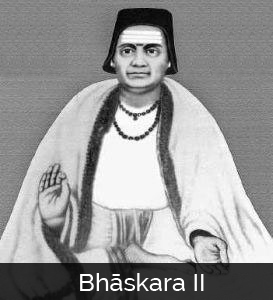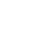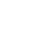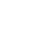Bhaskara II is a famous Indian mathematician. He also goes by the name of Bhaskara or Bhaskaracharya, which means Bhaskara the Teacher. Bhaskara is famous for a number of innovations in mathematics.

His knowledge of solving equations and number systems were at such a high level that it would take European mathematicians hundreds of years to attain this level.

Bhaskara is viewed as one of the greatest mathematicians of medieval India and his work has influenced both Indian and Islamic mathematicians as well as European ones.

### Early LifeNot a lot is known about Bhaskara’s life. It is known that he was born in 1114 A.D. near Bijjada Bida (present day Bijapur). His father was a famous astrologer and mathematician by the name of Mahesvara. It was Bhaskara’s father who taught his son all about mathematics and astrology. This was a common practice at the time as sons followed in their father’s footsteps. In fact, Bhaskara also taught mathematics to his son Loksamudra. In 1207, his son helped establish a school focused on the study of Bhaskara’s writings.

Bhaskara worked at the astronomical observatory at Ujjain and soon became the head of the facility.

This observatory was the top mathematical center in India and excellent mathematicians, such as Varahamihira and Brahmagupta, had also worked at the observatory.

Bhaskara, as did other Indian mathematicians, wrote in verse. He looked at various branches of mathematics, such as algebra, trigonometry, and calculus. He also studied and wrote about astronomy. In fact, half of his most famous work dealt with mathematical astronomy.

Bhaskara died in 1185 at Ujjain. His work was built on and developed after his death by another Indian mathematician, Madhava of Sangamagrama, as well as by mathematicians at the Kerala School. This work not only helped to expand Bhaskara’s work but it also expanded the development of calculus in India.

### His Writings

Bhaskara wrote a number of books but the work that has had the most influence in the mathematics field is the Siddhanta Siromani (Crown of Treatises). The book was written in 1150 A.D. when Bhaskara was thirty-six years old.

It has 1450 verses and is divided into four parts; although, sometimes the books are viewed as separate books. The titles of each section are Lilavati, Bijaganita, Grahagaṇita, and Goladhyaya. Each section deals with a different area of mathematics and astronomy.

Sometimes the last two books (Grahaganita and Goladhyaya) have been treated as the only two parts of the Siddhanta Siromani, while the first two books (Lilavati and Bijaganita) are viewed as two independent books.

### The Lilavati

The Lilavati focuses on arithmetic and according to a story that was written in a Persian translation of the book, the book was written for his daughter, who was named Lilavati. According to the story, Bhaskara made a horoscope of his daughter and found that her husband would die shortly after the couple were married.

The only way to prevent the death was to make sure that the marriage happened exactly at a specific time. To ensure that the marriage happened at the correct time, Bhaskara made a small hole in a cup and placed it in a pail filled with water.

Bhaskara had calculated how long it would take for the cup to fill and sink. He placed the cup in the water so that it would sink at the exact time Lilavati was supposed to get married. Bhaskara then warned Lilavati to stay away from the cup.

Lilavati couldn’t stay away and had to look at the device. While she was looking at the device, a pearl from her dress fell into the cup and blocked the hole.

As a result, the hour set for the marriage passed without the marriage taking place. As a result, Lilavati was widowed soon after her marriage took place. To make Lilavati feel better, Bhaskara wrote her a book about mathematics.

Lilavatihas thirteen chapters and covers a number of topics. The book deals with definitions and terms as well as looking at the properties of zero.

Bhaskara’s work with zero included dividing with zero and the rules of operations. Bhaskara also looked at negative numbers and surds in this book. Surds are simply square roots that are not whole numbers.

Bhaskara looked at different methods to perform calculations, such as multiplication and squaring, and he also wrote about the rule of three.

Bhaskara covered a number of other topics in Lilavati as well, such as trigonometry and mensuration, and even included a number of problems that readers of the book could work on. Bhaskara’s book was basically a textbook, so he included problems for the student to work through.

The work Bhaskara did in the book on indeterminate equations and integer solutions is the most important material in the book. The rules that Bhaskara wrote about were the same rules that European mathematicians would come up with almost five hundred years later.

Bhaskara was writing in the 12th century while European mathematicians “discovered” the rules in the 17th century. Bhaskara’s method of solving indeterminate equations was also an improvement over the methods worked out by Aryabhata.

### The Bijaganita

The Bijaganita focuses on algebra and has twelve chapters. In this book, Bhaskara wrote about his discovery that each positive number can have both a positive square root and a negative square root. He was the first person to realize this.

In addition to his work on positive and negative numbers, Bhaskara also looked methods to determine unknown quantities and did more work on the number zero.

He also improved on Aryabhata’s Kuttaka method for solving Diophantine and indeterminate equations. Bhaskara also looked at ways to expand upon some of the work done by Brahmagupta.

In addition to indeterminate equations, the book looks at quadratic, and simple equations as well as methods for evaluating surds.

Bhaskara’s method for finding the solution for Pell’s equation is considered to be very important. Bhaskara used the chakravala method to not only solve Pell’s equation but other indeterminate quadratic equations as well.

In both the Lilavatiand the Bihaganita, Bhaskara’s worked on dividing by zero. Bhaskara realized that when dividing one by a fraction, the smaller the fraction gets, the more pieces are created.

For example, 1 ÷ 1⁄2 = 2 and 1 ÷ 1⁄3 = 3; if you continue with smaller and smaller fractions, the results get bigger. Bhaskara came to the conclusion that if you divide one by zero, you will end up with an infinite number of pieces.

At first glance this might seem to be correct, but when looked at from the terms of multiplication, it becomes obvious that it is wrong. If n÷0=∞, then 0×∞=n. This would mean that all numbers are the same, which is obviously not true. The problem was that Indian mathematicians were not able to accept the fact that it is impossible to divide by 0.

The Ganitadhyaya deals with mathematical astronomy. Bhaskara based his astronomy on the earlier work by Aryabhata. Bhaskara viewed the solar system as being heliocentric (it revolves around the sun) and the planets have an elliptical orbit. Bhaskara also used the law of gravity that was proposed by Brahmagupta.

This book consists of twelve chapters and covers a variety of astronomical topics. Bhaskara looked at the mean longitudes and the true longitudes of the planets as well as latitudes. He also looked at both lunar and solar eclipses.

Bhaskara discussed the conjunction of the planets with each other and with fixed stars as well as with the orbits of both the Sun and the Moon. This book also examined the problems associated with diurnal rotation as well as syzygies. Syzygy is the alignment of three celestial bodies into an almost straight line.

Bhaskara, using only his naked eyes and without any sophisticated equipment, was able to calculate the length of time it takes the Earth to go around the Sun to within one minute of today’s calculation. Bhaskara calculated that it would take 365.2588 days for the Earth to orbit the Sun. Today’s calculation is 365.2596 days—a difference of one minute.

In the Goladhyaya, Bhaskara looked at the sphere. It is broken into thirteen chapters and covers areas such as the nature of a sphere and the armillary sphere. An armillary sphere is a model showing the globe. It used rings and hoops to depict the equator and the tropics.

Bhaskara also covered cosmography, geography and the seasons. He looked at planetary mean motion and methods for calculating ellipses and lunar crescents. He also discussed astronomical instruments and the difficulties involved with making astronomical calculations.

In addition, the book covers an epicycle model of the planets. An epicycle model means that some planets, for example, the sun and the moon, move in small circles. These small circles then orbit the Earth (or some other planetary body).

Both the Golahhyaya and the Ganitadhyaya show that Bhaskara had strong knowledge of trigonometry. He knew about the sine table and relationships between various trigonometric functions.

One of his discoveries in this book was spherical trigonometry. Bhaskara seems to have an actual interest in trigonometry and used it to calculate the sines of 18- and 36-degree angles.

Bhaskara wasalso the first to come up with the now famous solution for the equationssin(a + b) and sin(a – b). Bhaskara found that the solution for sin(a + b) is sin(a) cos(b) + cos(a) sin(b) and the solution for sin(a – b) is sin(a) cos(b) – cos(a) sin(b).

Bhaskara’s work shows that he was aware of differential calculus although he may not have recognized the usefulness of his research in this area. The beginnings of infinitesimal calculus and mathematical analysis can be seen in his work.

There is also evidence of the beginning ideas behind what is now known as Rolle’s theorem in Bhaskara’s work. Bhaskara showed that when a planet is at its farthest or at its closest from Earth, the equation of the centre vanishes.

The equation of the centre is the measure of the distance between where a planet is and where it is predicted to be given the assumption that its movement is uniform.

From this, Bhaskara concluded that at some point, the differential of the equation of the centre is equal to zero. This has hints of the general mean value theorem which is usually derived by using Rolle’s theorem.

Bhaskara also discovered spherical trigonometry. This is a field in spherical geometry and is very important in the fields of astronomy, geodesy, and navigation.

Bhaskara did a lot of important work in calculus and developed ways to use the principles of differential calculus to deal with problems in astronomy.

Even though Isaac Newton and Gottfried Leibniz are viewed as the founders of differential and integral calculus, there is a lot of evidence that shows Bhaskara developed a number of the principles of differential calculus and that he may have been the first to come up with both the derivative and the differential coefficient as well as differential calculus.

He also came up with the beginnings of infinitesimal calculus and made a number of contributions in the field of integral calculus.

Bhaskara had a lot of influence on Islamic mathematicians and there is evidence that Islamic mathematicians knew of Bhaskara’s work shortly after it was written. This influence can be seen in the writings of various Islamic mathematicians.

Bhaskara’s work probably was not known in Europe until around the end of the twelfth century although not directly.

It is more probable that Bhaskara’s work became known in Europe through the work done by Islamic mathematicians.European mathematicians would read the work of Islamic mathematicians who used Bhaskara’s work.

Some scholars have argued that Bhaskara’s work shows traces of Diophantus’s work but this is now seen as a misguided effort by scholars to claim that European mathematicians influenced a lot of work being done by non-Europeans.

The claim that Bhaskara’s work shows signs of being influenced by Diophantus can easily be disputed by the fact that Indian mathematicians have been studying what have become known as Diophantine equations for centuries before Diophantus was even born.

•History
•Science
•Geography
•Biography
•US History